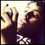# How Many A.Ps? Theory

Calvin Lin inspired me to this problem.In his problem Arithmetic Progressions.I tried to generalize the problem taking it as 'How many arithmetic progressions of length $t$ are there, such that the terms are integers from $1$ to $n$?'

I found that when common difference is $1$,total A.Ps will be $n-(t-1)$

When common difference is $2$,total A.Ps will be $n-2(t-1)$

The common difference will be continued till $[\frac {n}{t}]+1$

{ where$[\frac {n}{t}]$ is greatest integer contained in $\frac {n}{t}$ ]

Let $[\frac {n}{t}]=p$.

So I found that the total number of arithmetic progressions will be $n+(p+1)[2(n-t+1)-p(t-1)]$

For example $70+(7+1)[2(70-9+1)-7(9-1)]=70+8*[2*62-56]=614$

So the generalization formula will be $n+(p+1)[2(n-t+1)-p(t-1)]$

Please my friends if you find this useful Re-share and like.If you find any fault please tell me.Note by Kalpok Guha
4 years, 7 months ago

This discussion board is a place to discuss our Daily Challenges and the math and science related to those challenges. Explanations are more than just a solution — they should explain the steps and thinking strategies that you used to obtain the solution. Comments should further the discussion of math and science.

When posting on Brilliant:

• Use the emojis to react to an explanation, whether you're congratulating a job well done , or just really confused .
• Ask specific questions about the challenge or the steps in somebody's explanation. Well-posed questions can add a lot to the discussion, but posting "I don't understand!" doesn't help anyone.
• Try to contribute something new to the discussion, whether it is an extension, generalization or other idea related to the challenge.
• Stay on topic — we're all here to learn more about math and science, not to hear about your favorite get-rich-quick scheme or current world events.

MarkdownAppears as
*italics* or _italics_ italics
**bold** or __bold__ bold
- bulleted- list
• bulleted
• list
1. numbered2. list
1. numbered
2. list
Note: you must add a full line of space before and after lists for them to show up correctly
paragraph 1paragraph 2

paragraph 1

paragraph 2

[example link](https://brilliant.org)example link
> This is a quote
This is a quote
    # I indented these lines
# 4 spaces, and now they show
# up as a code block.

print "hello world"
# I indented these lines
# 4 spaces, and now they show
# up as a code block.

print "hello world"
MathAppears as
Remember to wrap math in $$ ... $$ or $ ... $ to ensure proper formatting.
2 \times 3 $2 \times 3$
2^{34} $2^{34}$
a_{i-1} $a_{i-1}$
\frac{2}{3} $\frac{2}{3}$
\sqrt{2} $\sqrt{2}$
\sum_{i=1}^3 $\sum_{i=1}^3$
\sin \theta $\sin \theta$
\boxed{123} $\boxed{123}$

Sort by:

There is a very simple way to obtain the total number of AP's of a fixed length $t$.

Hint: What can you say about $a_t - a_1$?

Staff - 4 years, 7 months ago

Thank you for you hint but sir can you please define $a_t$ and $a_1$ here?

- 4 years, 7 months ago

The first and last term of the arithmetic progression of length t.

Staff - 4 years, 7 months ago